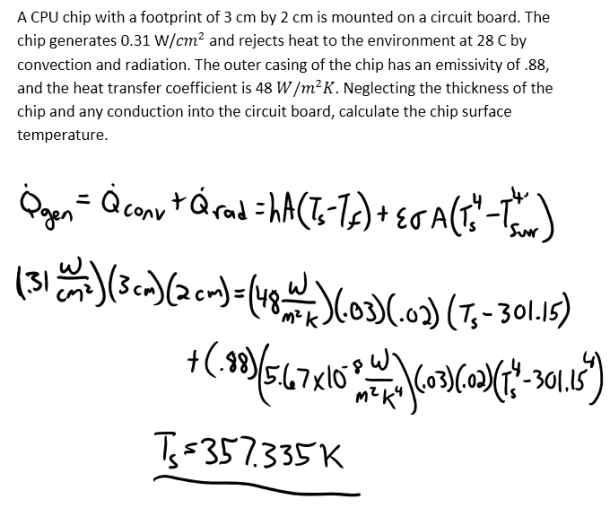heat and mass transfer problems engineering equations heat and mass transfer school homework engineering heat and mass transfer formulas heat and mass transfer solutions to heat and mass transfer problems full solution engineering problem solution heat and mass transfer math problems engineering equations heat and mass transfer school homework engineering solutions to heat and mass transfer formulas heat problems mass problem solutions to transfer problems full solution heat and mass transfer
heat and mass transfer problems engineering equations heat and mass transfer school homework engineering heat and mass transfer formulas heat and mass transfer solutions to heat and mass transfer problems full solution engineering problem solution heat and mass transfer math problems engineering equations heat and mass transfer school homework engineering solutions to heat and mass transfer formulas heat problems mass problem solutions to transfer problems full solution heat and mass transfer
Highalphabet Home Page heat and mass transfer problem solutions Heat and Mass Transfer Page
A CPU chip with a footprint of 3 cm by 2 cm is mounted on a circuit board. The chip generates 0.31 W/cm^2 and rejects heat to the environment at 28 C by convection and radiation. The outer casing of the chip has an emissivity of .88, and the heat transfer coefficient is 48 W/m^2 K. Neglecting the thickness of the chip and any conduction into the circuit board, calculate the chip surface temperature.A CPU chip with a footprint of 3 cm by 2 cm is mounted on a circuit board. The chip generates 0.31 W/cm^2 and rejects heat to the environment at 28 C by convection and radiation. The outer casing of the chip has an emissivity of .88, and the heat transfer coefficient is 48 W/m^2 K. Neglecting the thickness of the chip and any conduction into the circuit board, calculate the chip surface temperature.